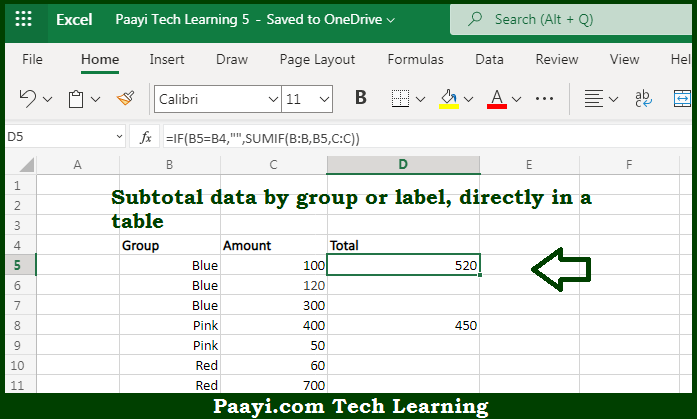# Learn How to SUM By Groups in Microsoft Excel

Written by | 0 Comments | 583 Views

In this article, you will learn how to COUNT various things in Microsoft Excel using a single/combination(s) of functions. You will also know how to SUM By Groups and see the generic formula.

SUM By Groups in Microsoft Excel

The main purpose of this formula is to subtotal data by group or label, directly in a table. Here we will learn how to sum by the group in Microsoft Excel. That implies, with the help of a formula based on the SUMIF function you can able to subtotal data by group or label, directly in a table. So, with the help of this formula, you can able to sum by the group in Microsoft Excel.

General Formula to SUM By Groups

=IF(A2=A1,"",SUMIF(A:A,A2,B:B))

The Explanation for the SUM By GroupsSo we know that with the help of the given formula above you can able to subtotal data by group or label, directly in a table. Here we will learn how to sum by a group in Microsoft Excel. As we know that the whole function of this formula is based on the IF function. It is used to test each value in column B to see if the same as the value in the "cell above". When values match, the formula returns nothing like this - (""). It should be noted that when values are different, the IF function calls the SUMIF function. So, with the help of this formula, you can able to subtotal data by group or label, directly in a table.